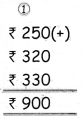Tamilnadu State Board New Syllabus Samacheer Kalvi 4th Maths Guide Pdf Term 3 Chapter 5 Money Ex 5.4 Textbook Questions and Answers, Notes.

## Tamilnadu Samacheer Kalvi 4th Maths Solutions Term 3 Chapter 5 Money Ex 5.4

Question 1.
Priya bought 20 balloons. If the cost of one balloon is ₹ 6, then find the cost of 20 balloons.
Cost of one ballon = ₹ 6
Cost of 20 ballon = 20 × 6
= ₹ 120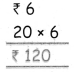Question 2.
Chinthamani bought 28 chocolate for her birthday. If the cost of a chocolate is ₹ 7 then find the total cost of 28 chocolates
cost of a chocolate = ₹ 7
cost of 28 chocolates = 28 × 7
= ₹ 196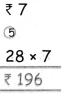Question 3.
Ashok purchased 9 decorative papers at the cost of ₹ 450 for his town festival. Find the cost of a decorative paper?
cost of 9 decorative papers = ₹ 450
cost of 1 decorative paper = 450 ÷ 9
= ₹ 50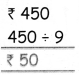Question 4.
Geethanjali bought 10 pencils at the cost of ₹ 70 from the supermarket. Find the cost of a pencil?
cost of 10 pencils = ₹ 70
cost of 1 pencil = 70 ÷ 10
= ₹ 7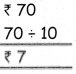Question 5.
Kuppan bought pencil and pen for ₹ 24.50 and ₹ 6.50 from his total amount ₹ 50. Find the total cost of things that he bought. Also find the balance amount?
Price of a pencil = ₹ 24.50
Price of a pen = ₹ 6.50
Sum = ₹ 24.50 + ₹ 6.50
= ₹ 31.00
Total amount = ₹ 50.00
Sum = ₹ 31.00
= ₹ 50.00 + ₹ 31.00
= ₹ 19.00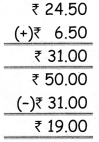Question 6.
A teacher went to the science exhibition with their student. The entry fee is ₹ 250 and the teacher bought some materials for the
school for ₹ 320, she had the balance amount of ₹ 330, find the amount that she had?